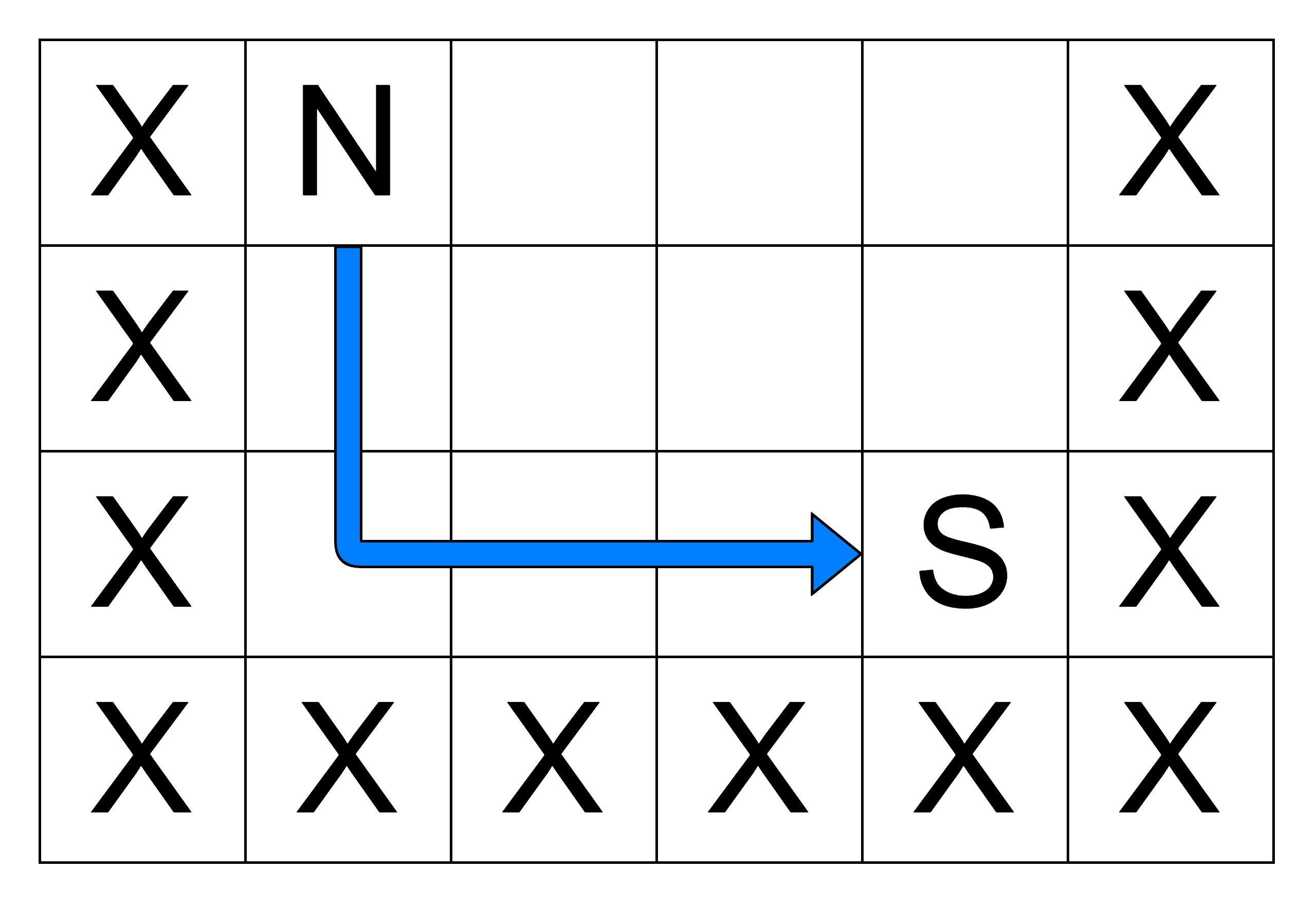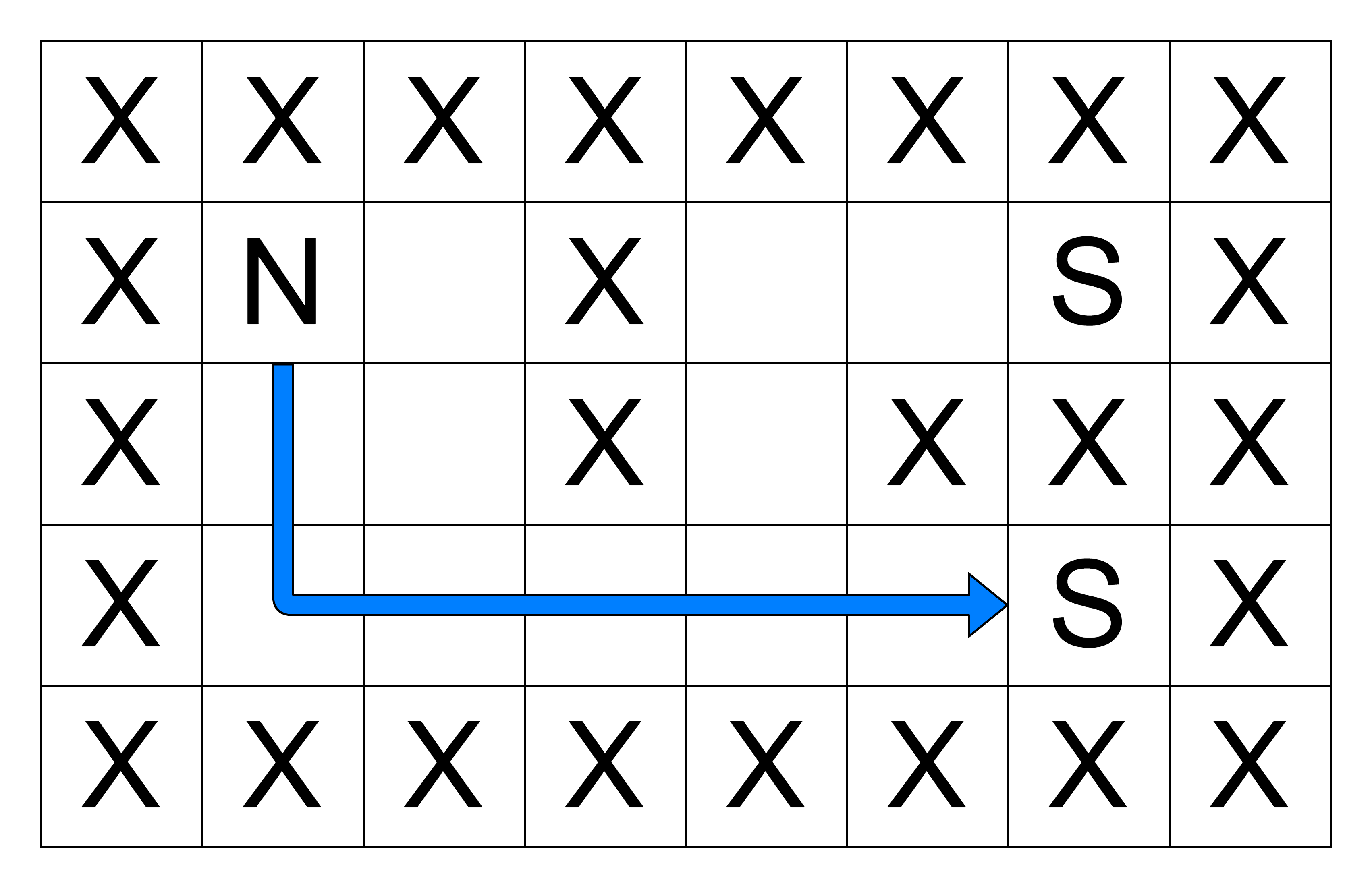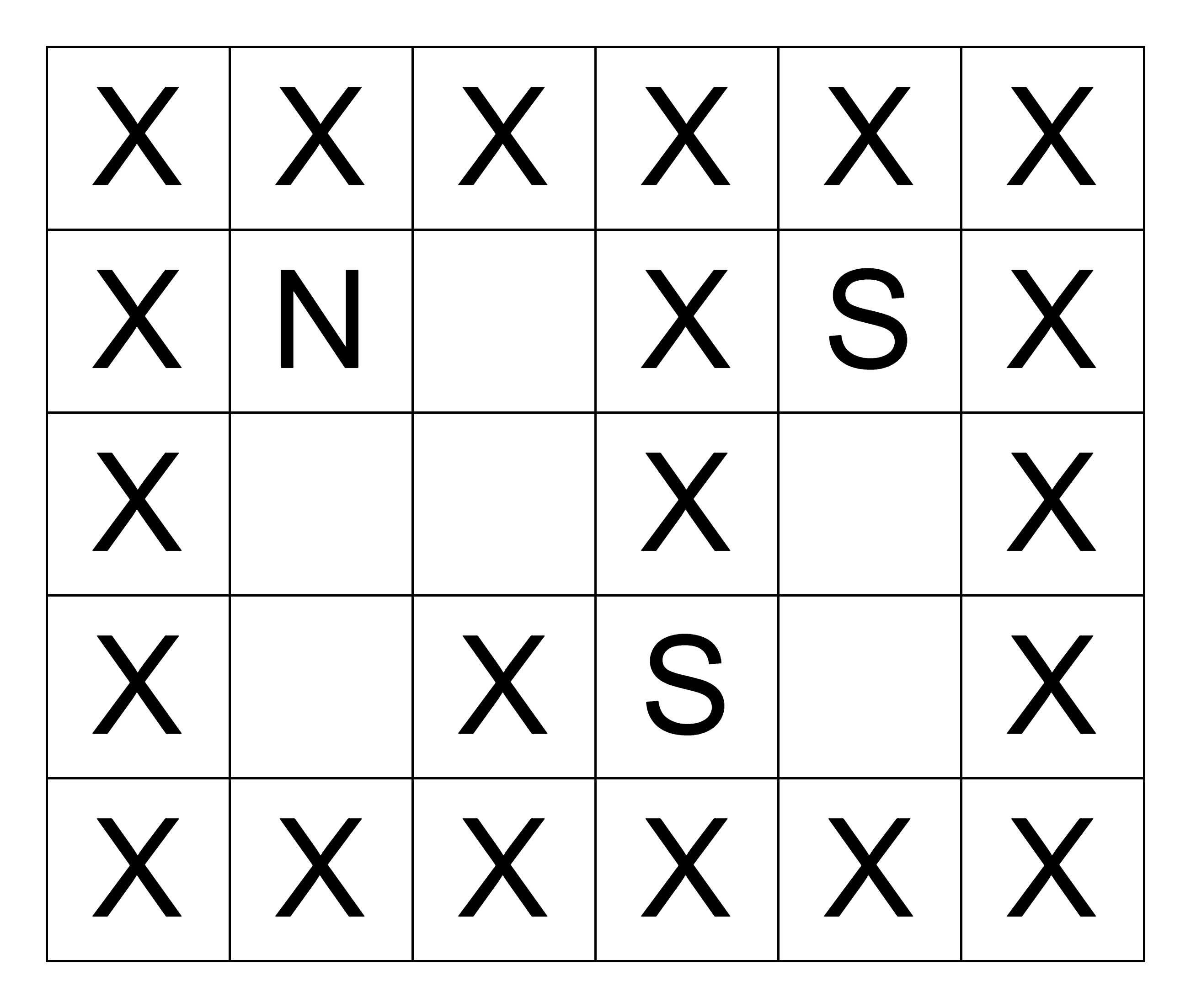New update is available. Click here to update.Close
Topic list
Path to the nearest service station
MEDIUM
15 mins3 upvotes
Graph
Matrices (2D Arrays)
Topics (Covered in this problem)
Problem solved
Skill meterMatrices (2D Arrays)
-
-Graph
-
-
Other topics
Problem solved
Skill meterStrings
-
--
-Sorting
-
-Binary Search
-
-Stacks & Queues
-
-Trees
-
-Dynamic Programming
-
-Greedy
-
-Tries
-
-Arrays
-
-SQL
-
-Binary Search Trees
-
-Heap
-
-Bit Manipulation
-
-
Solve problems & track your progress
Checkout your overall progress in every topic here
BecomeSensei
in DSA topics
Open the topic and solve more problems associated with it to improve your skills
Check out the skill meter for every topic
See how many problems you are left with to solve for cracking any stage. Score more than zero to get your progress counted.

# Path to the nearest service station

Medium0/80
Avg time to solve 15 mins
Success Rate 85 %Share3 upvotes

## Problem Statement

#### The Ninja can only travel to adjacent free cells from his current location, i.e., in the North, South, East, or West direction. Your task is to find the length of the shortest path through which the Ninja can get to a service station. If there is no such path available, return -1.

##### Example:
``````‘N’ = 4, ‘M’ = 6
Given ‘GRID’:
(Empty cells represent the character ‘O’)
````````````The Ninja can reach the nearest service station by moving two cells south and then three cells east. So, the answer is ‘5’.
``````
Detailed explanation ( Input/output format, Notes, Constraints, Images )#### Sample input 1:

``````2
5 8
X X X X X X X X
X N O X O O S X
X O O X O X X X
X O O O O O S X
X X X X X X X X
5 6
X X X X X X
X N O X S X
X O O X O X
X O X S O X
X X X X X X
``````

#### Sample output 1:

``````7
-1
``````

#### Explanation of sample input 1:

``````Test Case 1:
‘N’ = 5, ‘M’ = 8
Given ‘GRID’:
(Empty cells represent the character ‘O’)
````````````The Ninja can reach the nearest service station by moving two cells south and then five cells east. So, the answer is ‘7’.

Test Case 2:
‘N’ = 5, ‘M’ = 6
Given ‘GRID’:
(Empty cells represent the character ‘O’)
````````````As the Ninja cannot reach any service station because of obstacles, the answer is ‘-1’.
``````

#### Sample input 2:

``````2
4 5
X X X X X
X N O O O
X O O O S
X X X X X
3 4
N O S X
O O X X
S O X X
``````

#### Sample output 2:

``````4
2
``````AutoConsole